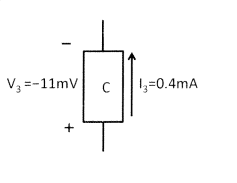# Does this obey passive sign convention? (Circuits)I thought passive sign convention is obeyed when you have a positive current flowing into the positive terminal of the element first. But how about this case? While it is drawn that way, the positive terminal is actually the NEGATIVE terminal because the voltage is negative, so you have to flip it.

Does this still count as obeying the passive sign convention then? In other words, POSITIVE current flows into POSITIVE terminal of NEGATIVE voltage.

Imagine you have a system that pumps water in a cycle. If the water goes up some hill in the process then it gains potential energy, and ,conversely, loses potential energy for when water flows downward. If the current flows from the negative end of the resistor to the positive, it has gained voltage, as if the water moved to a higher potential. Current can be thought of as water pressure in our imaginary system. If the water flows uphill to a higher potential then the water pressure will be less as energy was exerted to push the water, so likewise, current will be negative.

Simon Bridge
Homework Helper
The labeling appears ambiguous:
Does the + and - sign indicate the cathode and anode of the component, or which end is more positive than the other wrt to the voltage?

If the + end has the higher potential, the the voltage measured from - to + is 11.3mV and the current flows in the direction of the arrow through the component.

The usual convention is to draw arrows on components showing voltage and on wires showing current.

When applying kirkoffs laws we often have to guess the arrow directions at the start. If we guess wrong, then the magnitude that ends up associated with the arrow will be negative.

So the interpretation of the diagram depends on the context.